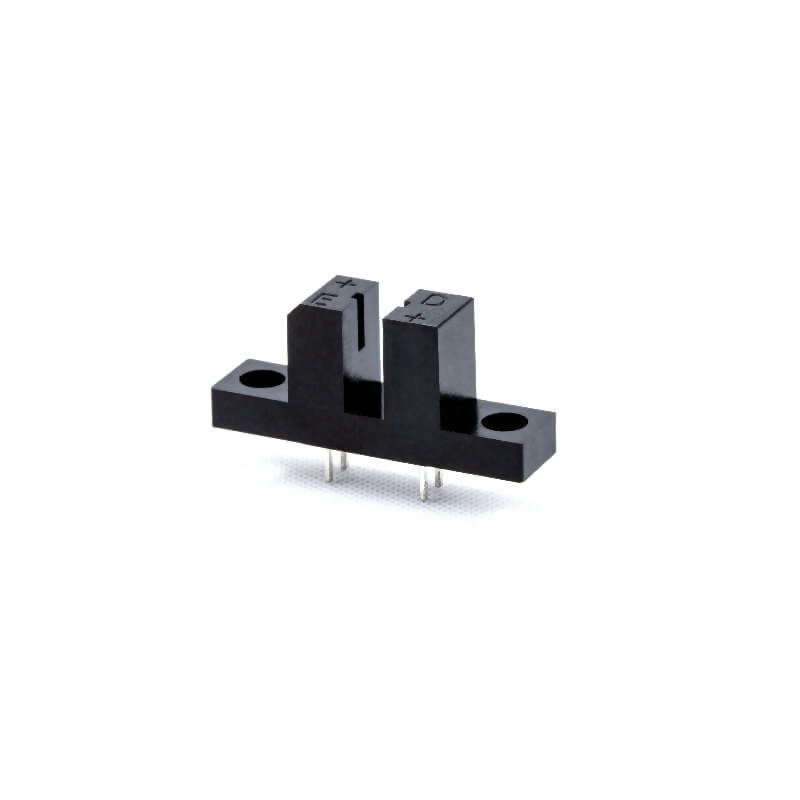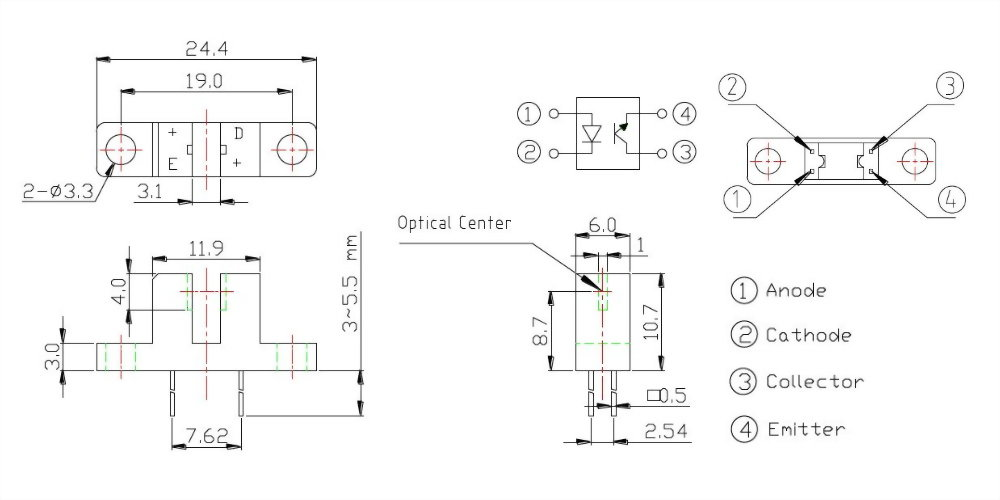## 槽寬3.1mm 雙耳紅外線光遮斷器

•DPI050100

 *型號: -- 請選擇 --DPI050100Absolute Maximum Rating（Ta=25°C）

 Item Symbol Rating Unit Input Power Dissipation Pd 75 mW Reverse Voltage VR 5 V Forward Current IF 30 mA Peak Forward Current IFP 0.1 A Output Collector Power Dissipation PC 75 mW Collector Current IC 20 mA C-E Voltage VCEO 30 V E-C Voltage VECO 5 V Operating Temperature Topr -40~+85 °C Storage Temperature Tstg -40~+100 °C Soldering Temperature   t=5 Sec Tsol 260 °C

Notes:

(*1) tw=100μsec , T=10 msec   (*2) t≦5 Sec

 Parameter Symbol Condition Min. Typ. Max. Unit Forward Voltage VF IF=20mA - 1.2 1.5 V Reverse Current IR VR=5V - - 10 μA Peak Wavelength λp IF=20mA 940 nm Dark Current Iceo VCE=20V - - 100 nA C-E Saturation Voltage VCE (sat) IC=0.5mA IF=20mA - - 0.4 V Light Current IC VCE=5V  IF=10mA 0.5 - - mA

Notes:

(*1) tw=100μsec , T=10 msec    (*2) t≦5 Sec

 輸入電流 (mA) 操作電壓 (V) 條件 10 5 VCE=5V   RD=470 ohm   RL=33K ohm

• 定位檢知
• 紙張檢知
• 信號檢知

• 結構簡單，具有多種安裝角度可選擇
• 外殼採用高抗張強度之工程塑膠製成，可避免安裝時產生破裂現象
• 用紅外線發射二極體和光電晶體配對組合，可減少可見光之干擾
• 符合 RoHS 標準
• 台灣製造
• 焊接流程完成後，請勿使用溶劑或類似物品清洗開關
• 使用水溶性助焊劑可能導致開關破損
• 由於公司政策是不斷的改善產品品質，因此規格書可能在沒有知會的情況下變更或修改，您可以透過本公司的業務人員取得最新資訊。在一般情況下，本公司所有產品都是依照規格書的標準條件生產
• 自動控制系統
• 自動化設備
• 掃描器
• 印表機
• 傳真機
• 影印機
• 印刷機械
• 滑鼠
• 安全系統
• 計數器
• 光學譯碼器
• 其他有關 定位檢知 、紙張檢知 、信號檢知之偵測